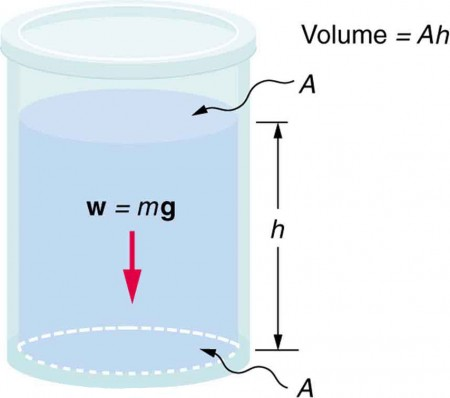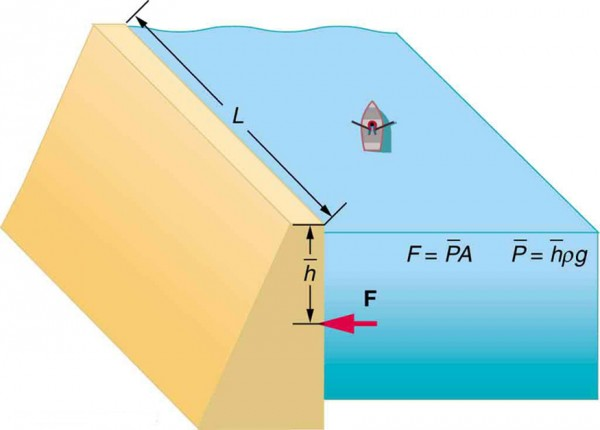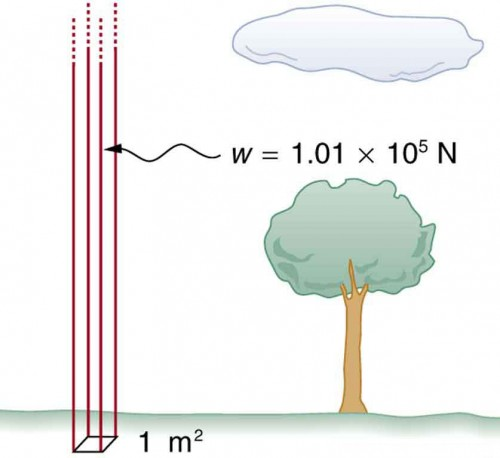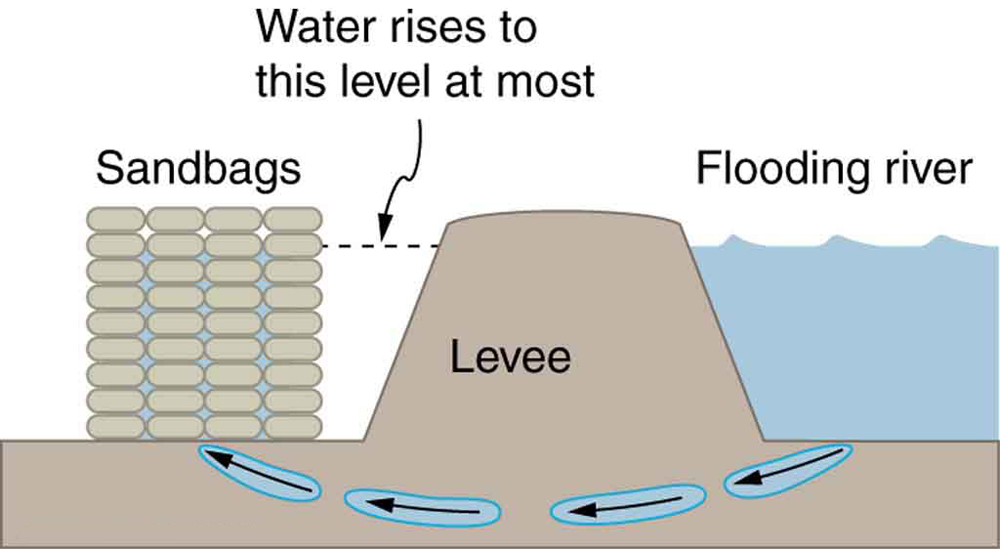Variation of Pressure with Depth in a Fluid

Learning Objectives

By the end of this section, you will be able to:

• Define pressure in terms of weight.
• Explain the variation of pressure with depth in a fluid.
• Calculate density given pressure and altitude.

If your ears have ever popped on a plane flight or ached during a deep dive in a swimming pool, you have experienced the effect of depth on pressure in a fluid. At the Earth’s surface, the air pressure exerted on you is a result of the weight of air above you. This pressure is reduced as you climb up in altitude and the weight of air above you decreases. Under water, the pressure exerted on you increases with increasing depth. In this case, the pressure being exerted upon you is a result of both the weight of water above you and that of the atmosphere above you. You may notice an air pressure change on an elevator ride that transports you many stories, but you need only dive a meter or so below the surface of a pool to feel a pressure increase. The difference is that water is much denser than air, about 775 times as dense. Consider the container in Figure 1.Figure 1. The bottom of this container supports the entire weight of the fluid in it. The vertical sides cannot exert an upward force on the fluid (since it cannot withstand a shearing force), and so the bottom must support it all.

Its bottom supports the weight of the fluid in it. Let us calculate the pressure exerted on the bottom by the weight of the fluid. That pressure is the weight of the fluid mg divided by the area A supporting it (the area of the bottom of the container):

$P=\frac{mg}{A}\\$.

We can find the mass of the fluid from its volume and density:

ρV.

The volume of the fluid V is related to the dimensions of the container. It is

V = Ah,

where A is the cross-sectional area and h is the depth. Combining the last two equations gives

$m=\rho Ah\\$.

If we enter this into the expression for pressure, we obtain

$P=\frac{\left(\rho{Ah}\right)g}{A}\\$.

The area cancels, and rearranging the variables yields

hρg.

This value is the pressure due to the weight of a fluid. The equation has general validity beyond the special conditions under which it is derived here. Even if the container were not there, the surrounding fluid would still exert this pressure, keeping the fluid static. Thus the equation hρg represents the pressure due to the weight of any fluid of average density ρ at any depth h below its surface. For liquids, which are nearly incompressible, this equation holds to great depths. For gases, which are quite compressible, one can apply this equation as long as the density changes are small over the depth considered. Example 2: Calculating Average Density: How Dense Is the Air? illustrates this situation.

Example 1. Calculating the Average Pressure and Force Exerted: What Force Must a Dam Withstand?

In Example 1. Calculating the Mass of a Reservoir from Its Volume, we calculated the mass of water in a large reservoir. We will now consider the pressure and force acting on the dam retaining water. (See Figure 2.) The dam is 500 m wide, and the water is 80.0 m deep at the dam. (a) What is the average pressure on the dam due to the water? (b) Calculate the force exerted against the dam and compare it with the weight of water in the dam (previously found to be 1.96 × 1013 N).Figure 2. The dam must withstand the force exerted against it by the water it retains. This force is small compared with the weight of the water behind the dam.

Strategy for (a)

The average pressure $\overline{P}\\$ due to the weight of the water is the pressure at the average depth $\overline{h}\\$ of 40.0 m, since pressure increases linearly with depth.

Solution for (a)

The average pressure due to the weight of a fluid is

$\overline{P}=\overline{h}rho g\\$.

Entering the density of water from Table 1 and taking $\overline{h}\\$ to be the average depth of 40.0 m, we obtain

$\begin{array}{lll}\overline{P}& =& \left(\text{40.0 m}\right)\left({\text{10}}^{3}\frac{\text{kg}}{{\text{m}}^{3}}\right)\left(9.80\frac{\text{m}}{{\text{s}}^{2}}\right)\\ & =& 3.92\times {\text{10}}^{5}\frac{\text{N}}{{\text{m}}^{2}}=\text{392 kPa.}\end{array}\\$

Strategy for (b)

The force exerted on the dam by the water is the average pressure times the area of contact:

$F=\overline{P}A\\$.

Solution for (b)

We have already found the value for $\overline{P}\\$. The area of the dam is A = 80.0 m x 500 m = 4.00 × 104 m2, so that

$\begin{array}{lll}F& =& \left(3.92\times {\text{10}}^{5}{\text{N/m}}^{2}\right)\left(4.00\times {\text{10}}^{4}{\text{m}}^{2}\right)\ & =& 1.57\times {\text{10}}^{\text{10}}\text{N.}\end{array}\\$

Discussion

Although this force seems large, it is small compared with the 1.96 × 1013 N weight of the water in the reservoir—in fact, it is only 0.0800% of the weight. Note that the pressure found in part (a) is completely independent of the width and length of the lake—it depends only on its average depth at the dam. Thus the force depends only on the water’s average depth and the dimensions of the dam, not on the horizontal extent of the reservoir. In the diagram, the thickness of the dam increases with depth to balance the increasing force due to the increasing pressure depth to balance the increasing force due to the increasing pressure.

Table 1. Densities of Various Substances
Substance $\rho \left({\text{10}}^{3}{\text{kg/m}}^{3}\text{or}\text{g/mL}\right)\\$ Substance $\rho \left({\text{10}}^{3}{\text{kg/m}}^{3}\text{or}\text{g/mL}\right)\\$ Substance $\rho \left({\text{10}}^{3}{\text{kg/m}}^{3}\text{or}\text{g/mL}\right)\\$
Solids Liquids Gases
Aluminum 2.7 Water (4ºC) 1.000 Air 1.29 × 10−3
Brass 8.44 Blood 1.05 Carbon dioxide 1.98 × 10−3
Copper (average) 8.8 Sea water 1.025 Carbon monoxide 1.25 × 10−3
Gold 19.32 Mercury 13.6 Hydrogen 0.090 × 10−3
Iron or steel 7.8 Ethyl alcohol 0.79 Helium 0.18 × 10−3
Lead 11.3 Petrol 0.68 Methane 0.72 × 10−3
Polystyrene 0.10 Glycerin 1.26 Nitrogen 1.25 × 10−3
Tungsten 19.30 Olive oil 0.92 Nitrous oxide 1.98 × 10−3
Uranium 18.70 Oxygen 1.43 × 10−3
Concrete 2.30–3.0 Steam (100º C) 0.60 × 10−3
Cork 0.24
Glass, common (average) 2.6
Granite 2.7
Earth’s crust 3.3
Wood 0.3–0.9
Ice (0°C) 0.917
Bone 1.7–2.0

Atmospheric pressure is another example of pressure due to the weight of a fluid, in this case due to the weight of air above a given height. The atmospheric pressure at the Earth’s surface varies a little due to the large-scale flow of the atmosphere induced by the Earth’s rotation (this creates weather “highs” and “lows”). However, the average pressure at sea level is given by the standard atmospheric pressure Patm, measured to be

$\text{1 atmosphere (atm)}={P}_{\text{atm}}=1.01\times {\text{10}}^{5}{\text{N/m}}^{2}=\text{101 kPa}\\$.

This relationship means that, on average, at sea level, a column of air above 1.00 m2 of the Earth’s surface has a weight of 1.01 × 105 N, equivalent to 1 atm. (See Figure 3.)Figure 3. Atmospheric pressure at sea level averages 1.01 × 105 Pa (equivalent to 1 atm), since the column of air over this 1 m2, extending to the top of the atmosphere, weighs 1.01 × 105.

Example 2. Calculating Average Density: How Dense Is the Air?

Calculate the average density of the atmosphere, given that it extends to an altitude of 120 km. Compare this density with that of air listed in Table 1.

Strategy

If we solve hρg for density, we see that

$\overline{\rho }=\frac{P}{\text{hg}}\\$.

We then take P to be atmospheric pressure, h is given, and g is known, and so we can use this to calculate $\overline{\rho}\\$.

Solution

Entering known values into the expression for $\overline{\rho}\\$ yields

$\overline{\rho }=\frac{1\text{.}\text{01}\times {\text{10}}^{5}{\text{N/m}}^{2}}{\left(\text{120}\times {\text{10}}^{3}\text{m}\right)\left(9\text{.}\text{80}{\text{m/s}}^{2}\right)}=8\text{.}\text{59}\times {\text{10}}^{-2}{\text{kg/m}}^{3}\\$.

Discussion

This result is the average density of air between the Earth’s surface and the top of the Earth’s atmosphere, which essentially ends at 120 km. The density of air at sea level is given in Table 1 as 1.29 kg.m3—about 15 times its average value. Because air is so compressible, its density has its highest value near the Earth’s surface and declines rapidly with altitude.

Example 3. Calculating Depth Below the Surface of Water: What Depth of Water Creates the Same Pressure as the Entire Atmosphere?

Calculate the depth below the surface of water at which the pressure due to the weight of the water equals 1.00 atm.

Strategy

We begin by solving the equation hρg for depth h:

$h=\frac{P}{\mathrm{\rho g}}\\$.

Then we take P to be 1.00 atm and ρ to be the density of the water that creates the pressure.

Solution

Entering the known values into the expression for h gives

$h=\frac{1\text{.}\text{01}\times {\text{10}}^{5}{\text{N/m}}^{2}}{\left(1\text{.}\text{00}\times {\text{10}}^{3}{\text{kg/m}}^{3}\right)\left(9\text{.}\text{80}{\text{m/s}}^{2}\right)}=\text{10}\text{.}3\text{m}\\$.

Discussion

Just 10.3 m of water creates the same pressure as 120 km of air. Since water is nearly incompressible, we can neglect any change in its density over this depth.

What do you suppose is the total pressure at a depth of 10.3 m in a swimming pool? Does the atmospheric pressure on the water’s surface affect the pressure below? The answer is yes. This seems only logical, since both the water’s weight and the atmosphere’s weight must be supported. So the total pressure at a depth of 10.3 m is 2 atm—half from the water above and half from the air above. We shall see in Pascal’s Principle that fluid pressures always add in this way.

Section Summary

• Pressure is the weight of the fluid mg divided by the area A supporting it (the area of the bottom of the container):
$P=\frac{\text{mg}}{A}\\$.
• Pressure due to the weight of a liquid is given by
$P=h\rho g\\$,

where P is the pressure, h is the height of the liquid, ρ is the density of the liquid, and g is the acceleration due to gravity.

Conceptual Questions

1. Atmospheric pressure exerts a large force (equal to the weight of the atmosphere above your body—about 10 tons) on the top of your body when you are lying on the beach sunbathing. Why are you able to get up?

2. Why does atmospheric pressure decrease more rapidly than linearly with altitude?

3. What are two reasons why mercury rather than water is used in barometers?

4. Figure 4 shows how sandbags placed around a leak outside a river levee can effectively stop the flow of water under the levee. Explain how the small amount of water inside the column formed by the sandbags is able to balance the much larger body of water behind the levee.Figure 4. Because the river level is very high, it has started to leak under the levee. Sandbags are placed around the leak, and the water held by them rises until it is the same level as the river, at which point the water there stops rising.

5. Why is it difficult to swim under water in the Great Salt Lake?

6. Is there a net force on a dam due to atmospheric pressure? Explain your answer.

7. Does atmospheric pressure add to the gas pressure in a rigid tank? In a toy balloon? When, in general, does atmospheric pressure not affect the total pressure in a fluid?

8. You can break a strong wine bottle by pounding a cork into it with your fist, but the cork must press directly against the liquid filling the bottle—there can be no air between the cork and liquid. Explain why the bottle breaks, and why it will not if there is air between the cork and liquid.

Problems & Exercises

1. What depth of mercury creates a pressure of 1.00 atm?

2. The greatest ocean depths on the Earth are found in the Marianas Trench near the Philippines. Calculate the pressure due to the ocean at the bottom of this trench, given its depth is 11.0 km and assuming the density of seawater is constant all the way down.

3. Verify that the SI unit of hρg is N/m2.

4. Water towers store water above the level of consumers for times of heavy use, eliminating the need for high-speed pumps. How high above a user must the water level be to create a gauge pressure of 3.00 × 105 N/m2?

5. The aqueous humor in a person’s eye is exerting a force of 0.300 N on the 1.10-cm2 area of the cornea. (a) What pressure is this in mm Hg? (b) Is this value within the normal range for pressures in the eye?

6. How much force is exerted on one side of an 8.50 cm by 11.0 cm sheet of paper by the atmosphere? How can the paper withstand such a force?

7. What pressure is exerted on the bottom of a 0.500-m-wide by 0.900-m-long gas tank that can hold 50.0 kg of gasoline by the weight of the gasoline in it when it is full?

8. Calculate the average pressure exerted on the palm of a shot-putter’s hand by the shot if the area of contact is 50.0 cm2 and he exerts a force of 800 N on it. Express the pressure in N/m2 and compare it with the 1.00 × 106 pressures sometimes encountered in the skeletal system.

9. The left side of the heart creates a pressure of 120 mm Hg by exerting a force directly on the blood over an effective area of 15.0 cm2. What force does it exert to accomplish this?

10. Show that the total force on a rectangular dam due to the water behind it increases with the square of the water depth. In particular, show that this force is given by $F=\rho {\mathrm{gh}}^{2}L/2\\$, where $rho\\$ is the density of water, h is its depth at the dam, and L is the length of the dam. You may assume the face of the dam is vertical. (Hint: Calculate the average pressure exerted and multiply this by the area in contact with the water. (See Figure 5.)

Glossary

pressure:
the weight of the fluid divided by the area supporting it

Selected Solutions to Problems & Exercises

1. 0.760 m

3. $\begin{array}{lll}{\left(h\rho g\right)}_{\text{units}}& =& \left(\text{m}\right)\left({\text{kg/m}}^{3}\right)\left({\text{m/s}}^{2}\right)=\left(\text{kg}\cdot {\text{m}}^{2}\right)/\left({\text{m}}^{3}\cdot {\text{s}}^{2}\right)\\ & =& \left(\text{kg}\cdot {\text{m/s}}^{2}\right)\left({\text{1/m}}^{2}\right)\\ & =& {\text{N/m}}^{2}\end{array}\\$.

5. (a) 20.5 mm Hg (b) The range of pressures in the eye is 12–24 mm Hg, so the result in part (a) is within that range

7. 1.09 × 103 N/m2

9. 24.0 N